# Percentage Calculator Tutorial

In this article as well as in the video below I go over an in-depth tutorial on how to use our percentage calculator as well as deriving the formulas shown for each of the calculations by hand.

## Breakdown of Calculations

Similar to the above video, screenshots of our percentage calculator are shown below and each calculation is discussed as well the formula derived.

## 'What percentage of' Calculation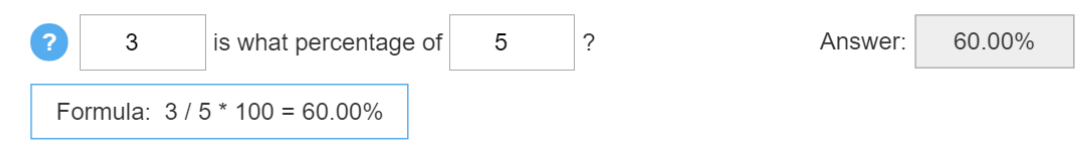In this example, the calculation solves the question: "3 is what percentage of 5?". This is the most basic percentage calculation and is given simply as the ratio of 3/5 in the form of a percentage, which is simply done by multiplying by 100. The idea behind this is to show what ratio or percentage 3 is of 5 or in other words "how much of 5 can 3 fit in". This is simply another way of stating the definition of a ratio and thus the resulting formula is simply:

3/5*100 and the answer is 60.00%.

## 'What number is a percentage of' Calculation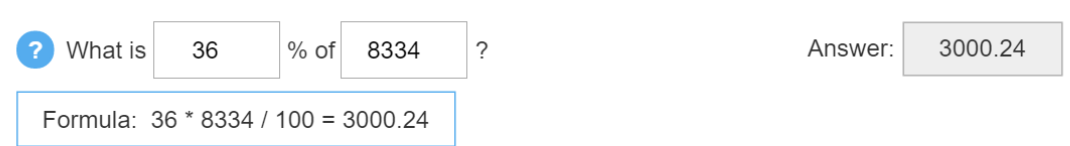In this example, the calculation solves the question: "What is 36% of 8334?". This calculation is very similar to the first calculation but now we are given the percentage and asked to solve for the value that would give this percentage. The best way to understand this is simply writing out the percentage as a ratio where 8334 is the denominator and then solving for the missing value as in the following equation:

X / 8334 = 36%

X = 36% * 8334 = 36/100 * 8334 = 3000.24

## 'A number is a percentage of' Calculation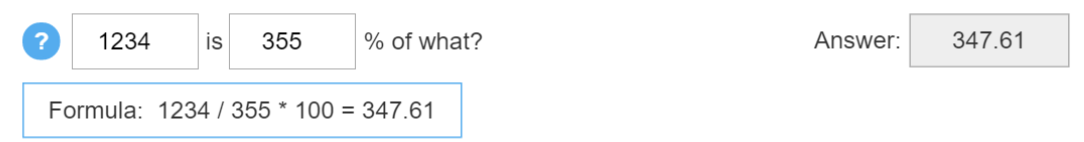In this example, the calculation solves the question: "1234 is 355% of what?". This calculation is once again similar to the first calculation but this time what is being calculated is the denominator of a ratio of percentage. Thus we can write out the ratio where the numerator is the value 1234 and then solve the denominator as shown below:

1234 / X = 355%

X = 1234 / 355% = 1234 / (355 / 100) = 347.61

## 'Increase from or Decrease from' Calculation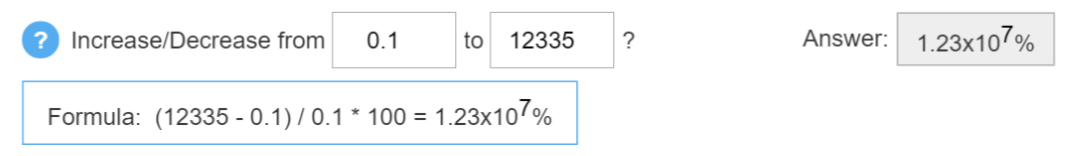In this example, the calculation solves the question: "Increase from 0.1 to 12335?". This calculation may seem a bit different than the above examples but in fact it is still similar since we will still write the formula as a ratio of two numbers. In this case the ratio is simply the increase from 0.1 to 12335 (which is just the difference between these two numbers) divided by 0.1 as shown in the steps below:

Increase / 0.1 = (12335 - 0.1) / 0.1 * 100 = 12,334,900 ≈ 1.23 x 107 %.

Note that the final answer is rounded up and written in Scientific Notation which to make sure the answer fits into the answer box. Scientific Notation is simply a number written as a multiply of 10 to the power of another number. In our case 1.23 x 107 simply means 1.23 * 10,000,000 = 12, 300, 000 which is thus a rounded up version of the actual answer of 12, 334,900. This is especially useful when dealing even bigger numbers such as 1.23x1022 which if written normally out would be 12,300,000,000,000,000,000,000 which would not fit into our small answer box. Similarly this is can be used for very small numbers as explained further in the homepage of our percentage calculator so make sure to check that out.

## 'Percentage of a mixed fraction' Calculation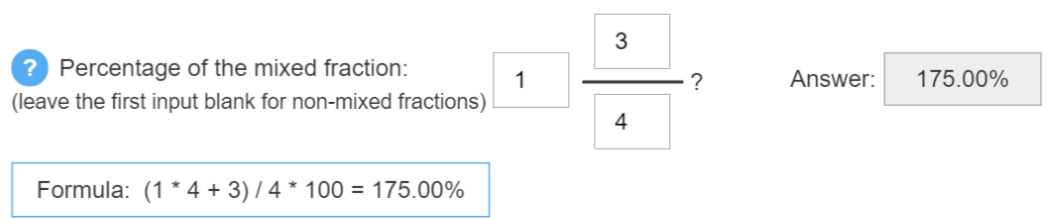In this example, the calculation solves the question: "Percentage of the mixed fraction: 1 3/4?". To idea behind mixed fractions is basically to simplify and make ratios or fractions more easily understandable. This is done by taking the number in front of the fraction as simply the number of complete fractions there are. Thus in our case since the fraction is 3/4 the number in front which is a 1 simply means that there is 1 whole fraction of 4/4. Thus the calculation, including factoring out the common denominator of 4, would simply be as follows:

1*4/4 + 3/4 = (1*4 + 3) / 4 = 175.00%

## Multiplication by a percentage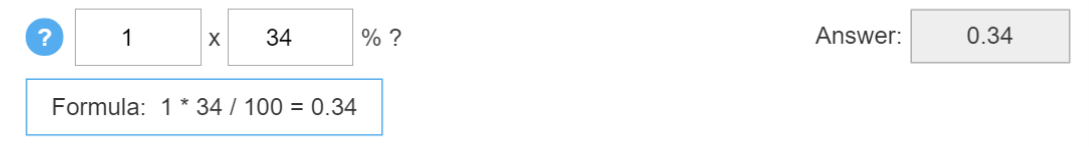In this example, the calculations solves the question "1 multiplied by 34%?". This is a very basic calculation and is simply involves writing 34% as a ratio of 34/100 as shown below:

1*34/100 = 0.34

## Division by a PercentageIn this example, the calculation solves the question "23 divided by 43?". This is a very similar to the multiplication by a percentage calculation and once again involves writing a percentage as a ratio with 100 as the denominator as shown below:

23 / (43 / 100) = 53.49

## Increase by a Percentage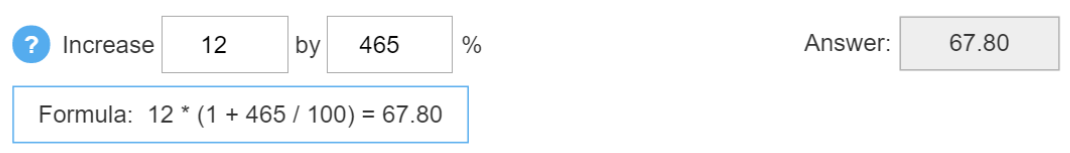In this example, the calculation solves the question "Increase 12 by 465%?". This is once again similar to the multiplication by a percentage calculation shown above but the only difference is that we simply add the number we are multiplying by, in this case 12. This is because the multiplication by a percentage is in fact an increase (or decrease if the percentage is less than 100%). The following steps below help illustrate this concept further:

Increase 12 by 465% = 12 + (465% of 12)

"465% of 12" is just the "what number is a percentage of" calculation and similarly we can write the ratio as follows:

• x / 12 = 465%
• x = 12 * 465% = 12 * 465/100 = the basic "multiplication by a percentage" calculation

Thus we can simply write the formula as follows, while factoring out the 12:

• 12 + 12*465% = 12 + 12*465/100 = 12*(1 + 465/100) = 67.80

## Decrease by a Percentage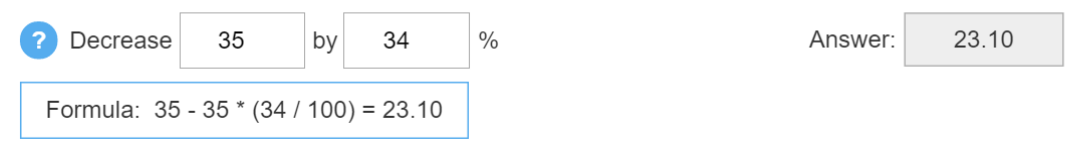In this example, the calculation solves the question "Decrease 35 by 34%?". This is the same calculation as the "increase by a percentage" calculation shown above but now we simply subtract instead of add as shown below:

Decrease 35 by 34% = 35 - 35*34% = 35 - 35*34/100 = 35(1 - 34/100) = 23.10